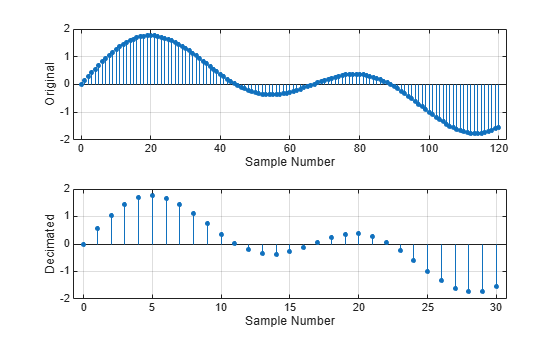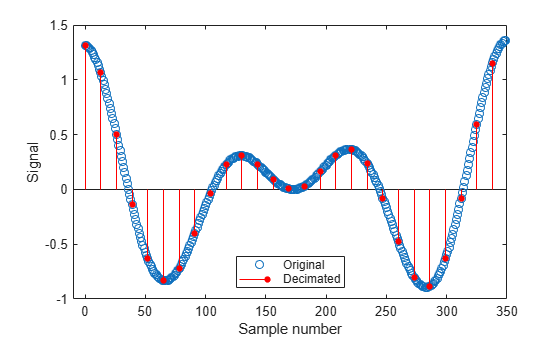Documentation

# decimate

Decimation — decrease sample rate by integer factor

## Syntax

``y = decimate(x,r)``
``y = decimate(x,r,n)``
``y = decimate(x,r,'fir')``
``y = decimate(x,r,n,'fir')``

## Description

example

````y = decimate(x,r)` reduces the sample rate of `x`, the input signal, by a factor of `r`. The decimated vector, `y`, is shortened by a factor of `r` so that ```length(y) = ceil(length(x)/r)```. By default, `decimate` uses a lowpass Chebyshev Type I infinite impulse response (IIR) filter of order 8.```

example

````y = decimate(x,r,n)` uses a Chebyshev filter of order `n`.```
````y = decimate(x,r,'fir')` uses a finite impulse response (FIR) filter designed using the window method with a Hamming window. The filter has an order of 30.```

example

````y = decimate(x,r,n,'fir')` uses an FIR filter of order `n`.```

## Examples

collapse all

Create a sinusoidal signal sampled at 4 kHz. Decimate it by a factor of four.

```t = 0:.00025:1; x = sin(2*pi*30*t) + sin(2*pi*60*t); y = decimate(x,4);```

Plot the original and decimated signals.

```subplot 211 stem(0:120,x(1:121),'filled','markersize',3) grid on xlabel 'Sample number',ylabel 'Original' subplot 212 stem(0:30,y(1:31),'filled','markersize',3) grid on xlabel 'Sample number',ylabel 'Decimated'```Create a signal with two sinusoids. Decimate it by a factor of 13 using a Chebyshev IIR filter of order 5. Plot the original and decimated signals.

```r = 13; n = 16:365; lx = length(n); x = sin(2*pi*n/153) + cos(2*pi*n/127); plot(0:lx-1,x,'o') hold on y = decimate(x,r,5); stem(lx-1:-r:0,fliplr(y),'ro','filled','markersize',4) legend('Original','Decimated','Location','south') xlabel('Sample number') ylabel('Signal')```The original and decimated signals have matching last elements.

Create a signal with two sinusoids. Decimate it by a factor of 13 using an FIR filter of order 82. Plot the original and decimated signals.

```r = 13; n = 16:365; lx = length(n); x = sin(2*pi*n/153) + cos(2*pi*n/127); plot(0:lx-1,x,'o') hold on y = decimate(x,r,82,'fir'); stem(0:r:lx-1,y,'ro','filled','markersize',4) legend('Original','Decimated','Location','south') xlabel('Sample number') ylabel('Signal')```The original and decimated signals have matching first elements.

## Input Arguments

collapse all

Input signal, specified as a vector.

Data Types: `double`

Decimation factor, specified as a positive integer. For better results when `r` is greater than 13, divide `r` into smaller factors and call `decimate` several times.

Data Types: `double`

Filter order, specified as a positive integer. IIR filter orders above 13 are not recommended because of numerical instability. The function displays a warning in those cases.

Data Types: `double`

## Output Arguments

collapse all

Decimated signal, returned as a vector.

Data Types: `double`

## Algorithms

Decimation reduces the original sample rate of a sequence to a lower rate. It is the opposite of interpolation. `decimate` lowpass filters the input to guard against aliasing and downsamples the result. The function uses decimation algorithms 8.2 and 8.3 from .

1. `decimate` creates a lowpass filter. The default is a Chebyshev Type I filter designed using `cheby1`. This filter has a normalized cutoff frequency of `0.8/r` and a passband ripple of 0.05 dB. Sometimes, the specified filter order produces passband distortion due to round-off errors accumulated from the convolutions needed to create the transfer function. `decimate` automatically reduces the filter order when distortion causes the magnitude response at the cutoff frequency to differ from the ripple by more than 10–6.

When the `'fir'` option is chosen, `decimate` uses `fir1` to design a lowpass FIR filter with cutoff frequency `1/r`.

2. When using the FIR filter, `decimate` filters the input sequence in only one direction. This conserves memory and is useful for working with long sequences. In the IIR case, `decimate` applies the filter in the forward and reverse directions using `filtfilt` to remove phase distortion. In effect, this process doubles the filter order. In both cases, the function minimizes transient effects at both ends of the signal by matching endpoint conditions.

3. Finally, `decimate` resamples the data by selecting every `r`th point from the interior of the filtered signal. In the resampled sequence (`y`), `y(end)` matches `x(end)` when the IIR filter is used, and `y(1)` matches `x(1)` when the FIR filter is used.

 Digital Signal Processing Committee of the IEEE® Acoustics, Speech, and Signal Processing Society, eds. Programs for Digital Signal Processing. New York: IEEE Press, 1979.